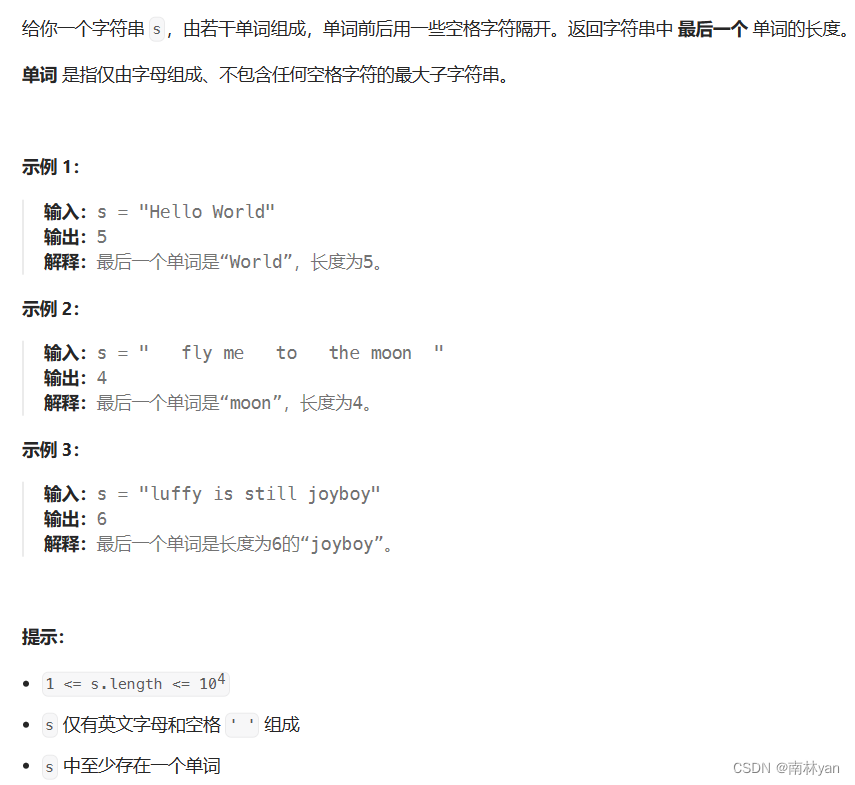# leetcode：58. 最后一个单词的长度int lengthOfLastWord(char * s)

``````int lengthOfLastWord(char * s){
//定位到最后一个单词的最后一个字符
int size = strlen(s);
int index = size - 1;
while (s[index] == ' ')
{
index--;
}

//设置长度变量n
int n = 0;
while (index >= 0 && s[index] != ' ')
{
index--;
n++;
}

return n;
}``````

第二种思路：

``````int lengthOfLastWord(char* s)
{
int n = 0;
int index = 0;
while (s[index] != '\0')//从前向后遍历字符串
{
if (s[index] != ' ')
{
if (index > 0 && s[index - 1] == ' ')//判断前一个字符是否为空格字符
{
n = 0;//新单词，重置长度变量
}
n++;
}
index++;
}
return n;
}``````

### 网站公告